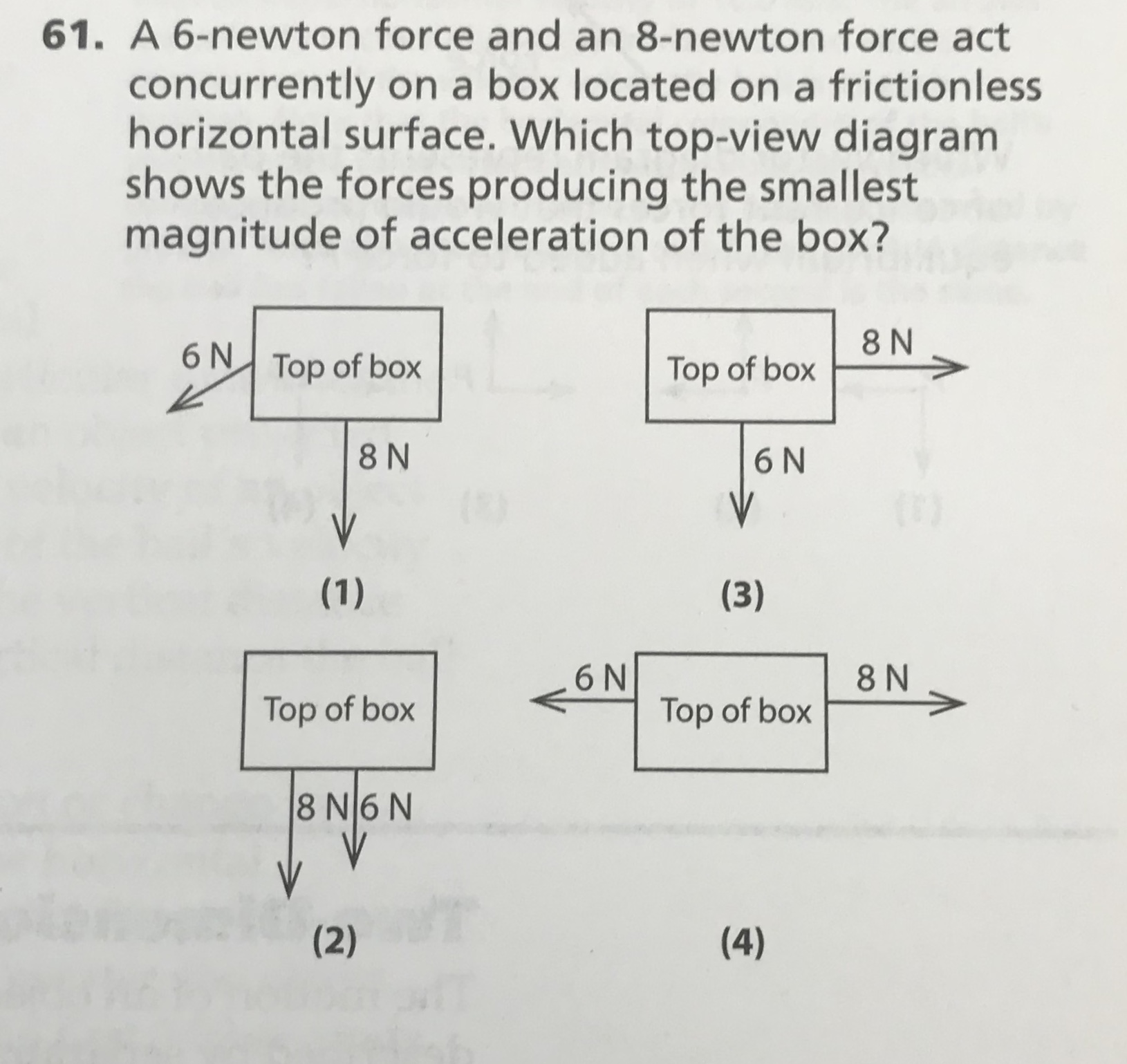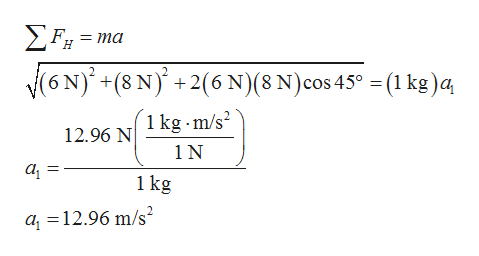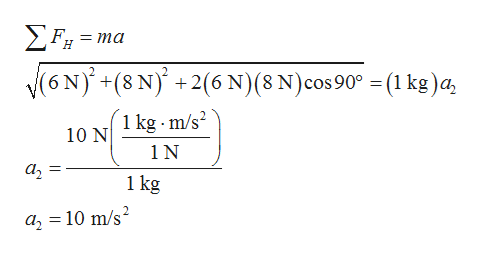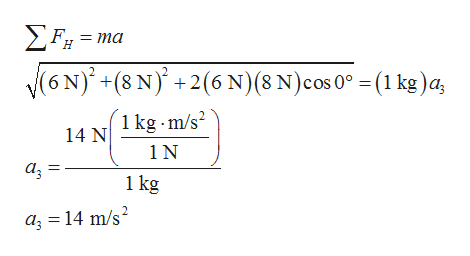# 61. A 6-newton force and an 8-newton force actconcurrently on a box located on a frictionlesshorizontal surface. Which top-view diagramshows the forces producing the smallestmagnitude of acceleration of the box?8 N6 NTop of boxTop of box8 N6NV(1)(3)6NTop of box8 NTop of box8 N6 N(2)(4)IN

Question
496 viewshelp_outlineImage Transcriptionclose61. A 6-newton force and an 8-newton force act concurrently on a box located on a frictionless horizontal surface. Which top-view diagram shows the forces producing the smallest magnitude of acceleration of the box? 8 N 6 N Top of box Top of box 8 N 6N V (1) (3) 6N Top of box 8 N Top of box 8 N6 N (2) (4) IN fullscreen
check_circle

Step 1

(1)

Consider the mass of the box be m as a unit mass that is 1 kg, the angle of 6 N force from the horizontal be θ.

Apply Newton’s second law of motion along the horizontal on the box, consider both the forces are at 45o, and solve for the acceleration of the box given in the first diagram.help_outlineImage TranscriptioncloseΣ, - ma (6N)+(8 N)+2(6 N)(8 N) cos 45° = (1 kg)a 1 kg m/s 12.96 N 1N 1 kg a 12.96 m/s fullscreen
Step 2

(2)

Here, both the forces are perpendicular to each other, so the angle between these forces are 90o. Apply Newton’s second law of motion along the horizontal on the box, and solve for the acceleration of the box given in the second diagram.help_outlineImage TranscriptioncloseF 3D та Н V6NY+(8 N)+2(6 N) (8 N) cos 90" = (1 kg) a 1 kg m/s 10 N 1N а, 1 kg а, 3 10 m/s? fullscreen
Step 3

(3)

Here, both the forces are in same direction, so the angle between these forces is 0o. Thus, apply Newton’s second law of motion alo...help_outlineImage TranscriptioncloseΣ-ma 6N +(8 N)2(6 N)(8 N) cos 0° - (1 kg)4, 1 kg m/s2 14 N 1N 1 kg a 14 m/s2 fullscreen

### Want to see the full answer?

See Solution

#### Want to see this answer and more?

Solutions are written by subject experts who are available 24/7. Questions are typically answered within 1 hour.*

See Solution
*Response times may vary by subject and question.
Tagged in

### Kinematics